High School Algebra - Quadratic Equations: Homework Help Chapter Exam

Exam Instructions:

Choose your answers to the questions and click 'Next' to see the next set of questions. You can skip questions if you would like and come back to them later with the yellow "Go To First Skipped Question" button. When you have completed the practice exam, a green submit button will appear. Click it to see your results. Good luck!

Page 1

Question 1 1. Use the quadratic formula to find the solutions to the equation below.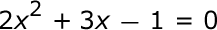Question 3 3. Find the roots of the equation below.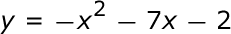Page 2

Question 7 7. Solve this inequality.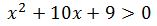Page 3

Question 11 11. What is the b-value of the quadratic equation below?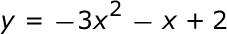Question 12 12. Rewrite the quadratic below in standard form: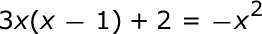Question 13 13. Solve the following inequality.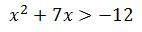Question 14 14. What are the zeros in the following quadratic equation?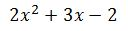Page 4

Question 18 18. Which of the following are the solutions to the equation below?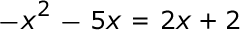Question 20 20. Find the roots of the equation.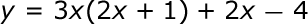Page 5

Question 22 22. Which statement is true about the inequality below?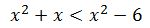Question 24 24. Solve the equation.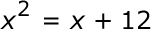High School Algebra - Quadratic Equations: Homework Help Chapter Exam Instructions

Choose your answers to the questions and click 'Next' to see the next set of questions. You can skip questions if you would like and come back to them later with the yellow "Go To First Skipped Question" button. When you have completed the practice exam, a green submit button will appear. Click it to see your results. Good luck!

Support